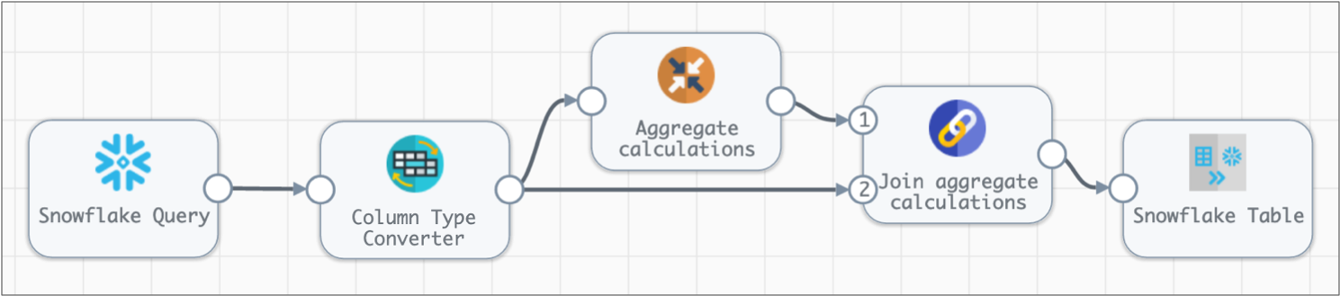# Aggregate

The Aggregate processor performs aggregate calculations, such as Sum or Median, across the incoming data. To perform calculations on subsets of a batch, you can specify columns to group by.

For example, you can use the Aggregate processor to calculate the sum of all purchases in a batch grouped by state, and to write the results to a `State_Total` output column in each row.

When you configure the Aggregate processor to perform a calculation, you specify the aggregation column, aggregate function, and output column to use. You can specify multiple sets of calculations to perform. You can optionally specify columns to group by for the calculations.

Important: Rows generated by the Aggregate processor include the output columns and any specified group-by columns. All other columns from the incoming row are dropped.

## Processing and Generated Rows

The Aggregate processor can perform one or more aggregate calculations on data in a batch. For each calculation that you want to perform, you specify the aggregate function, the column to use for the calculation, and an output column for the results.

By default, the processor performs calculations using all of the incoming data. To perform calculations on subsets of data, specify one or more columns to group by.

Rows generated by the Aggregate processor include the output columns and any specified group-by columns. All other columns from the incoming row are dropped.

### Example

Say you want to know the number of transactions and the total for those transactions for each state. To do this, you configure the processor as follows:
• Use the `COUNT` function and output the results to a `StateCount` column.
• Use the `SUM` function with the `Total` column, and output the results to a `StateTotal` column.
• Set the processor to group by the `State` column.

Let's say a batch contains the following data:

TransactionID StoreID State Total
0032355 35 MD 230.40
0016433 20 IL 90.50
0016434 20 IL 489.23
0032356 35 MD 63.50
0032357 35 MD 150.49
The Aggregate processor produces two output rows, one for the state of Maryland and the other for the state of Illinois. The processor writes the results of the aggregate calculations to the `StateCount` and `StateTotal` columns in each row as follows:
State StateCount StateTotal
MD 3 444.39
IL 2 579.73

## Joining Aggregate Data

Rows generated by the Aggregate processor include the output columns and any specified group-by columns. All other columns from the incoming row are dropped.

If you want to perform aggregate calculations, but do not want to discard the rest of the data, you can perform the aggregate calculations in a separate branch in the pipeline, and then merge the results back into the data.

For example, the following pipeline converts a few column data types before passing the data to the Aggregate processor. Then, the Join processor joins the aggregate calculations with the data from the Column Type Converter processor.## Aggregate Functions

You can use the following aggregate functions in an Aggregate processor:

• Average - Returns the average of the values in a group.
• Count - Returns the number of items in a group.
• Count Distinct - Returns the number of unique items in a group.
• Max - Returns the maximum value of a group.
• Median - Returns the median of the values of a group.
• Min - Returns the minimum value of a group.
• Mode - Returns the most frequent value within a group.
• Standard Deviation - Returns the standard deviation of a value from the group. Standard deviation uses a sample of a group rather than the full population.
• Sum - Returns the sum of the values of a group.
• Variance - Returns a sample variance of non-NULL rows in a group.
• Custom - Returns the results of a custom aggregate calculation.

## Configuring an Aggregate Processor

Configure an Aggregate processor to perform aggregate calculations across incoming data.
1. On the General tab, configure the following properties:
General Property Description
Name Stage name.
Description Optional description.
Cache Data Caches processed data.
2. On the Aggregate tab, configure the following properties:
Aggregate Property Description
Aggregations The aggregate calculations to perform. Configure the following properties:
• Aggregate Function - The aggregation function to use in the calculation.

Default is Average.

• Aggregate Column - The column to use in the aggregate calculation. You can enter the column name or select a column from preview data.

Not available for all aggregate functions.

• Calculation - Aggregate calculation to use. Available when using Custom Calculation as the aggregate function.
• Output Column - The column for the results of the calculation.